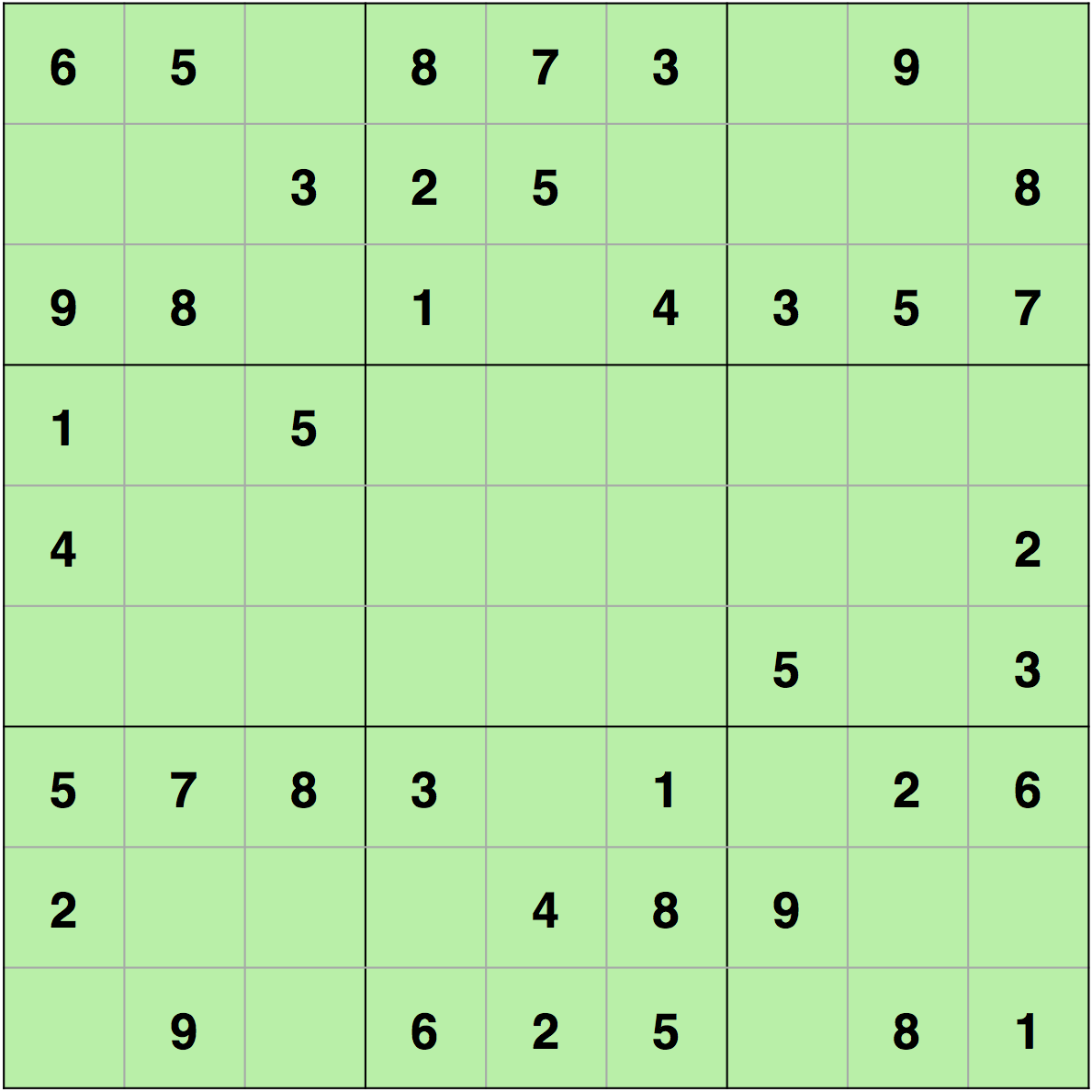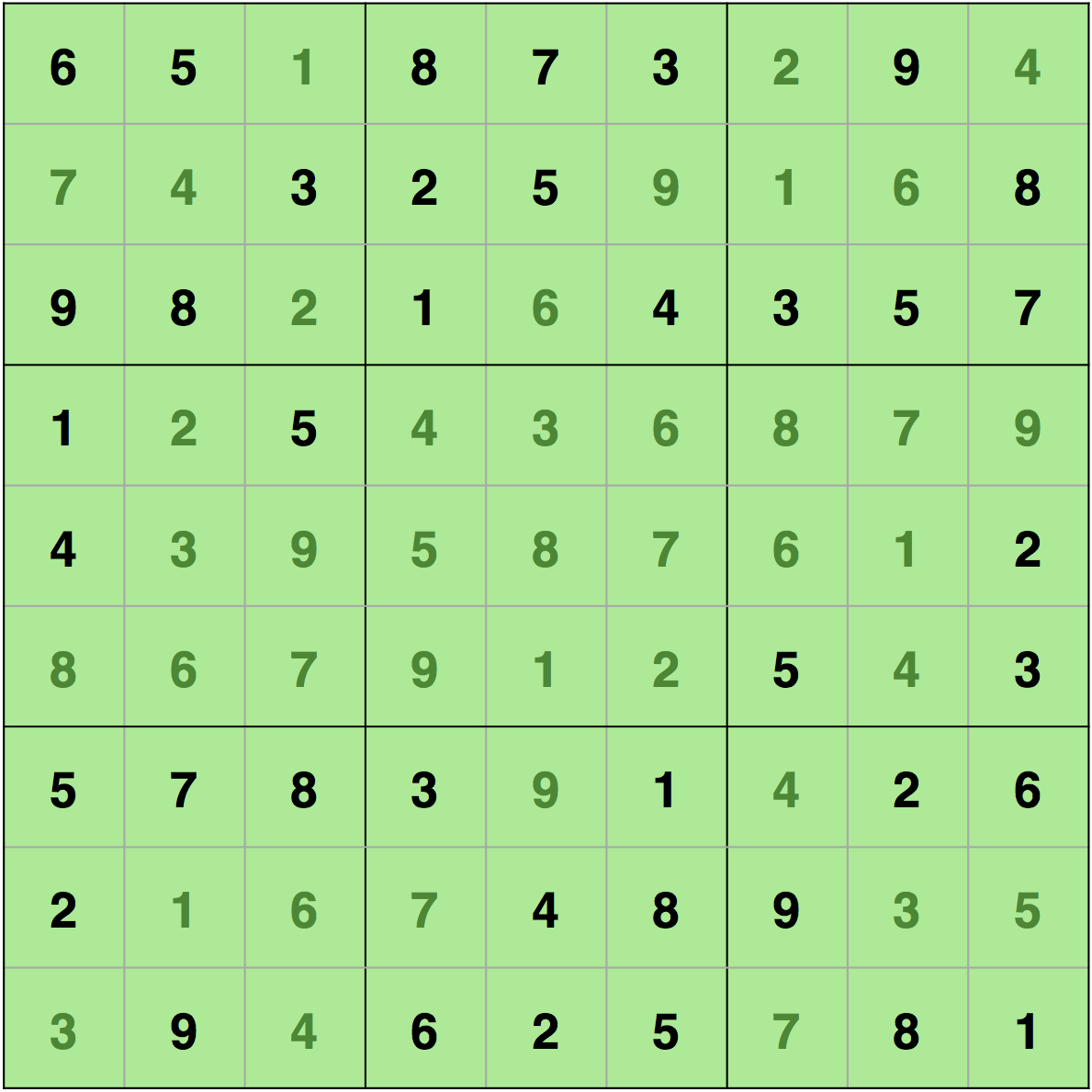# 代码实践：如何用 C、Java 和 Python 中的回溯求解数独问题？1. 任何一行都多次包含某个相同的数字；
2. 任何一列都多次包含某个相同的数字；
3. 任何一个 3×3 的子矩阵都多次包含某个相同的数字。

• 如果没有未分配的单元格，那么数独已被求解，我们将返回 true；
• 否则，我们将用 1 到 9 之间的数字填写未分配的单元格，以便在任何行、列或 3×3 子矩阵中都没有冲突；
• 现在，我们将尝试填写下一个未分配的单元格，如果这个操作成功，那么我们将返回 true；
• 否则，我们会返回并修改用来填写单元格的数字。如果没有满足需求的数字，那么我们将返回 false，因为这个数独没有解；

## C 语言

#include <stdio.h> #define SIZE 9 // 数独问题int matrix = {    {6,5,0,8,7,3,0,9,0},    {0,0,3,2,5,0,0,0,8},    {9,8,0,1,0,4,3,5,7},    {1,0,5,0,0,0,0,0,0},    {4,0,0,0,0,0,0,0,2},    {0,0,0,0,0,0,5,0,3},    {5,7,8,3,0,1,0,2,6},    {2,0,0,0,4,8,9,0,0},    {0,9,0,6,2,5,0,8,1}}; // 该方法用于打印数独void print_sudoku(){    int i,j;    for(i=0;i<SIZE;i++)    {        for(j=0;j<SIZE;j++)        {            printf("%d\t",matrix[i][j]);        }        printf("\n\n");    }} // 该方法用于检查是否还有未赋值的单元格// 如果有未赋值的单元格// 那么这个方法将相应地修改行和列的值int number_unassigned(int *row, int *col){    int num_unassign = 0;    int i,j;    for(i=0;i<SIZE;i++)    {        for(j=0;j<SIZE;j++)        {            // 单元格未被赋值            if(matrix[i][j] == 0)            {                // 修改行和列的值                *row = i;                *col = j;                // 有一个或多个未赋值的单元格                num_unassign = 1;                return num_unassign;            }        }    }    return num_unassign;} // 该方法用于检查是否可以填写一个// 值到对应的单元格中int is_safe(int n, int r, int c){    int i,j;    // 检查行    for(i=0;i<SIZE;i++)    {        // 某个单元格具有相同值        if(matrix[r][i] == n)            return 0;    }    // 检查列    for(i=0;i<SIZE;i++)    {        // 某个单元格的值等于 i        if(matrix[i][c] == n)            return 0;    }    // 检查子矩阵    int row_start = (r/3)*3;    int col_start = (c/3)*3;    for(i=row_start;i<row_start+3;i++)    {        for(j=col_start;j<col_start+3;j++)        {            if(matrix[i][j]==n)                return 0;        }    }    return 1;} // 该方法用回溯来求解数独问题int solve_sudoku(){    int row;    int col;    // 如果所有单元格都被赋值了的，那么数独问题已经通过引用被求解了；    // 因为 number_unassigned 将修改    if(number_unassigned(&row, &col) == 0)        return 1;    int n,i;    //1 到 9 之间的数字    for(i=1;i<=SIZE;i++)    {        // 是否可以将 i 赋值给单元格        // 单元格是 matrix[row][col]        if(is_safe(i, row, col))        {            matrix[row][col] = i;            // 回溯            if(solve_sudoku())                return 1;            // 如果我们不能继续求解这个问题            // 重新赋值单元            matrix[row][col]=0;        }    }    return 0;} int main(){    if (solve_sudoku())        print_sudoku();    else        printf("No solution\n");    return 0;}

## Java 语言

class Sudoku{     private static final int SIZE = 9;    private static int[][] matrix = {        {6,5,0,8,7,3,0,9,0},        {0,0,3,2,5,0,0,0,8},        {9,8,0,1,0,4,3,5,7},        {1,0,5,0,0,0,0,0,0},        {4,0,0,0,0,0,0,0,2},        {0,0,0,0,0,0,5,0,3},        {5,7,8,3,0,1,0,2,6},        {2,0,0,0,4,8,9,0,0},        {0,9,0,6,2,5,0,8,1}    };     private static void printSudoku()    {        for(int i=0;i<SIZE;i++)        {            for(int j=0;j<SIZE;j++)            {                System.out.print(matrix[i][j]+"\t");            }            System.out.println("");        }    }     // 该方法用于检查是否还有未赋值的单元格    // 如果有未赋值的单元格    // 那么这个方法将相应地修改行和列的值    private static int[] numberUnassigned(int row, int col)    {        int numunassign = 0;        for(int i=0;i<SIZE;i++)        {            for(int j=0;j<SIZE;j++)            {                // 单元格未被赋值                if(matrix[i][j] == 0)                {                    // 修改行和列的值                    row = i;                    col = j;                    // 有一个或多个未赋值的单元格                    numunassign = 1;                    int[] a = {numunassign, row, col};                    return a;                }            }        }        int[] a = {numunassign, -1, -1};        return a;    }     // 该方法用于检查是否可以填写一个    // 值到对应的单元格中    private static boolean isSafe(int n, int r, int c)    {        // 检查行        for(int i=0;i<SIZE;i++)        {            // 某个单元格具有相同值            if(matrix[r][i] == n)                return false;        }        // 检查列        for(int i=0;i<SIZE;i++)        {            // 某个单元格的值等于 i            if(matrix[i][c] == n)                return false;        }        // 检查子矩阵        int row_start = (r/3)*3;        int col_start = (c/3)*3;        for(int i=row_start;i<row_start+3;i++)        {            for(int j=col_start;j<col_start+3;j++)            {                if(matrix[i][j]==n)                    return false;            }        }        return true;    }     // 该方法用回溯来求解数独问题    private static boolean solveSudoku()    {        int row=0;        int col=0;        int[] a = numberUnassigned(row, col);        // 如果所有单元格都被赋值了的，那么数独问题已经通过引用被求解了；        // 因为 number_unassigned 将修改行和列的值        if(a == 0)            return true;        // 用 1 到 9 之间的数字        row = a;        col = a;        for(int i=1;i<=SIZE;i++)        {            // 是否可以将 i 赋值给单元格            // 单元格是 matrix[row][col]            if(isSafe(i, row, col))            {                matrix[row][col] = i;                // 回溯                if(solveSudoku())                    return true;                // 如果我们不能继续求解这个问题                // 重新赋值单元                matrix[row][col]=0;            }        }        return false;    }     public static void main(String[] args)    {        if (solveSudoku())            printSudoku();        else            System.out.println("No solution");    }}

## Python

SIZE = 9#数独问题#值为 0 的单元格为空单元格matrix = [    [6,5,0,8,7,3,0,9,0],    [0,0,3,2,5,0,0,0,8],    [9,8,0,1,0,4,3,5,7],    [1,0,5,0,0,0,0,0,0],    [4,0,0,0,0,0,0,0,2],    [0,0,0,0,0,0,5,0,3],    [5,7,8,3,0,1,0,2,6],    [2,0,0,0,4,8,9,0,0],    [0,9,0,6,2,5,0,8,1]]#该方法用于打印数独def print_sudoku():    for i in matrix:        print (i) #该方法用于检查是否还有未赋值的单元格#如果有未赋值的单元格#那么这个方法将相应地修改行和列的值def number_unassigned(row, col):    num_unassign = 0    for i in range(0,SIZE):        for j in range (0,SIZE):            #单元格未被赋值            if matrix[i][j] == 0:                row = i                col = j                num_unassign = 1                a = [row, col, num_unassign]                return a    a = [-1, -1, num_unassign]    return a#该方法用于检查是否可以填写一个#值到对应的单元格中def is_safe(n, r, c):    #检查行    for i in range(0,SIZE):        #某个单元格具有相同值        if matrix[r][i] == n:            return False    #检查列    for i in range(0,SIZE):        #某个单元格有相同的值        if matrix[i][c] == n:            return False    row_start = (r//3)*3    col_start = (c//3)*3;    #检查子矩阵    for i in range(row_start,row_start+3):        for j in range(col_start,col_start+3):            if matrix[i][j]==n:                return False    return True #该方法用回溯来求解数独问题def solve_sudoku():    row = 0    col = 0    #如果所有单元格都被赋值了的，那么数独问题已经通过引用被求解了；    #因为 number_unassigned 将修改行和列的值    a = number_unassigned(row, col)    if a == 0:        return True    row = a    col = a    #1 到 9 之间的数字    for i in range(1,10):        #是否可以将 i 赋值给单元格        #单元格是 matrix[row][col]        if is_safe(i, row, col):            matrix[row][col] = i            #回溯            if solve_sudoku():                return True            #如果我们不能继续求解这个问题            #重新赋值单元            matrix[row][col]=0    return False if solve_sudoku():    print_sudoku()else:    print("No solution")

## 代码说明

print_sudoku() ——这只是一个打印矩阵的函数。

number_unassigned ——该函数用于查找某个空单元格，并设置变量“行”和“列”的值等于该单元格的索引值。在 C 语言中，我们使用指针来修改传递（通过引用传递）给该函数的变量 (row, col) 的值。在 Java 和 Python 中，我们返回一个包含这些值的数组（或 Python 列表）。所以，这个函数告诉我们是否有未分配的单元格。如果有未分配的单元格，这个函数也会告诉我们该单元格的索引。

is_safe(int n, int r, int c) ——该函数检查是否可以将值“n”放入单元格 (r, c) 中。我们首先检查“r”行中是否有值为“n”的单元格（ if(matrix[r][i] == n) ）。然后，我们再检查“c”列中是否有值为“n”的单元格（ if(matrix[i][c] == n) ）。最后，我们检查子矩阵。 (r/3)*3 给出了行 r 的起始索引。例如，如果“r”的值是 2，则它在从 (0,0) 开始的子矩阵中。同样，我们可以得到起始列的值是 (c/3)*3 。因此，如果一个单元格是 (2,2) ，那么这个单元格就在一个从 (0,0) 开始的子矩阵中，我们可以通过 (c/3)*3 和 (r/3)*3 得到这个值。在得到起始索引之后，我们可以轻松地遍历子矩阵，以检查是否可以将值“n”放入该子矩阵中。

solve_sudoku() ——实际上，这才是使用回溯求解数独的函数。我们首先使用 number_unassigned 函数检查是否有未赋值的单元格，如果没有未赋值的单元格，那么数独问题已求解。number_unassigned 函数也会给出空单元格的索引。因此，如果有空单元格，我们将尝试用 1 到 9 之间的数值来填写此单元格。我们将使用 is_safe 来检查是否可以在该单元格中填写某个特定的值。在找到某个值之后，我们将尝试使用 solve_sudoku 求解剩余的数独问题。如果这个值不能求解剩余问题，我们将返回并尝试将单元格 matrix[row][col]=0; 赋其他值。循环尝试单元格中的其他值。

https://learnworthy.net/solving-sudoku-with-backtracking

## 评论2019 年 09 月 30 日 10:28